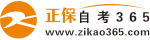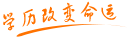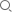#24小时客服：010-82335555# 自考“C语言程序设计”模拟试题三

2007年05月28日    来源：   字体：   打印

一、单项选择题

1. 设int x=1，y=1；表达式（！x++ || y——）的值是_________

A.0     B. 1     C. 2     D.-1

2.main（ ）

{  int n；

（ n=6*4，n+6），n*2；

printf（“n=%d\n”，n）；

}   此程序的输出结果是______________.

A.30    B.  24     C.  60      D.  48

3.若有如下定义，则__________是对数组元素的正确的引用。

int  a ， *p ；

p=a ；   p=a ；   p=a ；   p=a ；

A.  *&a     B.  a      C.  *（p+10）      D. *p

4.设整型变量n的值为2，执行语句“n+=n-=n*n；”后，n的值是__________

A.  0     B.  4    C.  - 4     D.  2

5.以下不能正确定义二维数组的语句是___________

A . int a={{1}，{2}}；     B. int a[]={1，2，3，4}；

C. int a={{1}，2，3}；      D. int a[]={{1，2}，{3，4}}；

6. 程序段的功能是将变量 u 、s中的最大值赋给变量t .

A.  if（u>s） t=u；t=s；       B.  t=u；   if（t ）  t=s；

C.  if（u>s） t=s；else t=u；    D .  t=s； if（u ）  t=u；

7.下列程序段的输出结果是           .

void main（）

{   int k；

for （k=1；k<5；k++〉

{  if（k%2！=0）

printf（“#”）；

else

printf（“*”） ；  }

}

A. #*#*      B . *#*#    C.  ##       D. 以上都不对

8.设变量定义为 int a={1，4，7}，*p=&a， 则*p的值是（35）    .

A. &a    B. 4       C. 7       D. 1

9.能正确表示a和b同时为正或同时为负的逻辑表达式是_________.

A.a>=0||b>=0）&&（a<0 ||b<0）      B.（a>=0 && b>=0）&&（a<0 && b<0）

C.（a+b>0） &&（a+b<=0）            D. a*b>0

10.C语言中，合法的字符型常数是_________.

A.‘A’        B.“A”        C. 65        D. A

11.设有数组定义：char array[]=“China”； 则数组所占的空间是________.

A.4个字节      B.5个字节      C.6个字节        D.7个字节

12.若变量c为char类型，能正确判断出c为小写字母的表达式是_______.

A.‘a’<=c<=‘z’

B. （c>=‘a’） || （c<=‘z’）

C.（‘a’<=c） and （‘z’>=c）

D. （c>=‘a’） && （c<=‘z’）

13.设有定义：long x=-123456L； 则以下能够正确输出变量x值的语句是_______.

A.printf（“x=%d\n”，x）          B.printf（“x=%ld\n”，x）

C.printf（“x=%8dl\n”，x）        D.printf（“x=%LD\n”，x）；

14.下列关于指针定义的描述，___________是错误的。

A.指针是一种变量，该变量用来存放某个变量的地址值的。

B.指针变量的类型与它所指向的变量类型一致。

C.指针变量的命名规则与标识符相同。

D.在定义指针时，标识符前的“*”号表示后面的指针变量所指向的内容。

15.已知：int x；int y；下列______是合法的。

A.&x      B.&（x+3）     C.&5       D .&y

16.指出下面正确的输入语句__________.

A.  scanf（“a=b=%d”，&a，&b）；

B. scanf（“%d，%d”，&a，&b）；

C.  scanf（“%c”，c）；

D. scanf（“% f%d\n”，&f）；

17.C语言中以追加方式打开一个文件应选择_______________参数。

A.“r”                  B.“w”

C.“rb”                 D.“a”

18.Break语句的正确的用法是（    ）。

A. 无论在任何情况下，都中断程序的执行，退出到系统下一层。

B. 在多重循环中，只能退出最靠近的那一层循环语句。

C. 跳出多重循环。

D. 只能修改控制变量。

19. 为表示关系x≥y≥z，应使用C语言表达式

A.（x>=y）&&（y>=z）    B. （x>=y） AND （y>=z）

C.（x>=y>=z）           D. （x>=z）&（y>=z）

20.以下可以作为C语言合法整数的是________.

A.1010B    B. 0368      C.0Xffa          D. x2a2

21.在函数中默认存储类型说明符的变量应该是________存储类型。

A.内部静态    B. 外部      C.自动          D. 寄存器

二、判断题

1.  C 语言源程序文件通过了编译、连接之后，生成一个后缀为 .EXE 的文件。 （           ）

2. 在 C 程序中，函数既可以嵌套定义，也可以嵌套调用。（        ）

3. 在 C 程序中，APH 和aph 代表不同的变量。（        ）

4. 表达式0195 是一个八进制整数。（        ）

5. 表达式_ya是不合法的C语言标识符。（        ）

6. C程序总是从main（ ）函数的第一条语句开始执行的。（      ）

7. A-=7等价于a=a-7.  （        ）

8. 利用fclose函数关闭已打开的文件。（        ）

9. 数组名可以作为参数进行传递。（        ）

10. 执行printf（“%x”，12345）；输出 12345 .（        ）

三、填空

1.C语言的预处理语句以__________开头。

2.表达式7+8>2 && 25 %5的结果是_______________________.

3.下列程序段是从键盘输入的字符中统计数字字符的个数，用换行符结束循环。

int  n=0， ch；

ch=getchar（ ）；

while（ __________________ ）

{  if （ ________________ ）  n++；

c=getchar（ ）；                  }

4.C语言中putchar（c ） 函数的功能是_________________________________.

5.int *p 的含义是___________________________________________.

6.定义fp为文件型指针变量的定义方法为_______________________.

7.数组 int  a；共定义了____________个数组元素。

四、读出程序

1. 改正下列程序中不正确的语句。

main（）

{ int a=8 ， b=1；

a=a+b；

b=a*b；

printf（“%d， %d”，a，b）；    }

2.写出下列程序的运行结果。

fun（int a， int b）

{ if（a>b） return （a）；

Else  return （b）；  }

main（）

{ int x=3，y=8，z=6，r；

r=fun（fun（x，y），2*z）；

printf（“%d\n”，r）；  }

3. 改正下列程序中不正确的语句。

main（）

{ int n ；

float s=1.0；

for（n=10；n>1；n- -）

s=s+1/n；

printf（“%6.1f\n”，s）；  }

4. 写出下列程序的运行结果。

main（）

{ int n；

For（n=3； n<=10； n++）

{ if（n%5= =0） break；

Printf（“%d”，n）； }            }

5.写出下列程序的运行结果。

#include “stdio.h”

main（）

{ int a[]={1，2，3，-4，5}；

int m，n，*p；

p=&a； p=&a；

m=*（p+2）；

n=*（p+4）；

printf（“%d  %d  %d  ”，*p，m，n）；  }

五、编程序

1.编程计算下列表达式：s=1！+2！+3！+4！+……+10！

2.从键盘上输入a与n的值，计算sum=a+aa+aaa+aaaa+…（共n项）的和。例a=2，n=4，则sum=2+22+222+2222.

3.求3X3矩阵的主对角线元素之和。

4.从键盘上输入10个数存入一维数组中，求这10数中的最大值与最小值并输出。

5.从键盘上输入若干个字符存入文件write.txt中，遇到回车键输入结束。（用“\n”表示回车键）

• 热门专业
• 报名咨询• 最新问答
• 热门问答